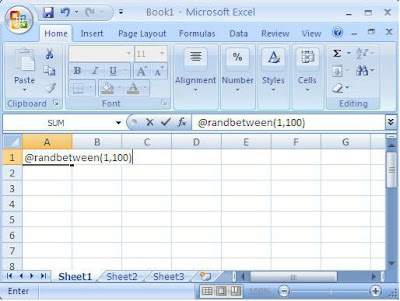## @randbetween(1,100)

Rolling dice, flipping coins, drawing cards or straws, drawing slips of paper out of a hat. All methods to generate random results. Here’s one more:

@randbetween(x,y)

To Create a Random Number: In a blank cell of any spreadsheet program, type @randbetween(x,y) where x equals the smallest number you want to use and y equals the largest. In other words, typing the function @randbetween(1,100) will generate a number between 1 and 100.Recalculate the Number: Press the “F9” key to generate a new number.

Change a Random Number to a Value: Note that any time you make a change to any cell in your spreadsheet, the program recalculates all the formulas on the sheet. That means the random number will recalculate every time a change is made to the spreadsheet. If you want to make your random value permanent, move to the cell with your @RANDBETWEEN function and press F2 (EDIT), then F9 (CALC).

Compatibility: This formula will work in Excel, Quattro Pro and Lotus 123. Have a different spreadsheet program? Give it a shot.

Microsoft Purists: Some of you may be thinking that Excel formulas begin with an equal sign, so the formula should be: “=randbetween(1,100)” True enough. Go ahead. But I’ve been using spreadsheet software for a long time (Lotus 123). We old timers know that spreadsheet formulas are “supposed” to begin with “@” and we also know that formulas beginning with an equal sign won’t work in Quattro Pro and Lotus. Because Microsoft is special.